#Function Repository Resource:

# BilliardPolygon

Show the trajectory of a ball bouncing in a regular n-sided polygon

Contributed by: Robert Dickau, Eric W. Weisstein
 ResourceFunction["BilliardPolygon"][sides,pos,θ,t] gives the trajectory of a ball in a regular n-sided polygon starting from position pos with an angle θ for t steps.

## Details and Options

Mathematical billiards consists of a ball bouncing with no friction against the sides of a plane or higher-dimensional object, in this case, a regular polygon. The trajectories show interesting behavior related to dynamical systems theory.

## Examples

### Basic Examples

 In:=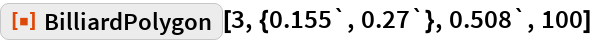Out=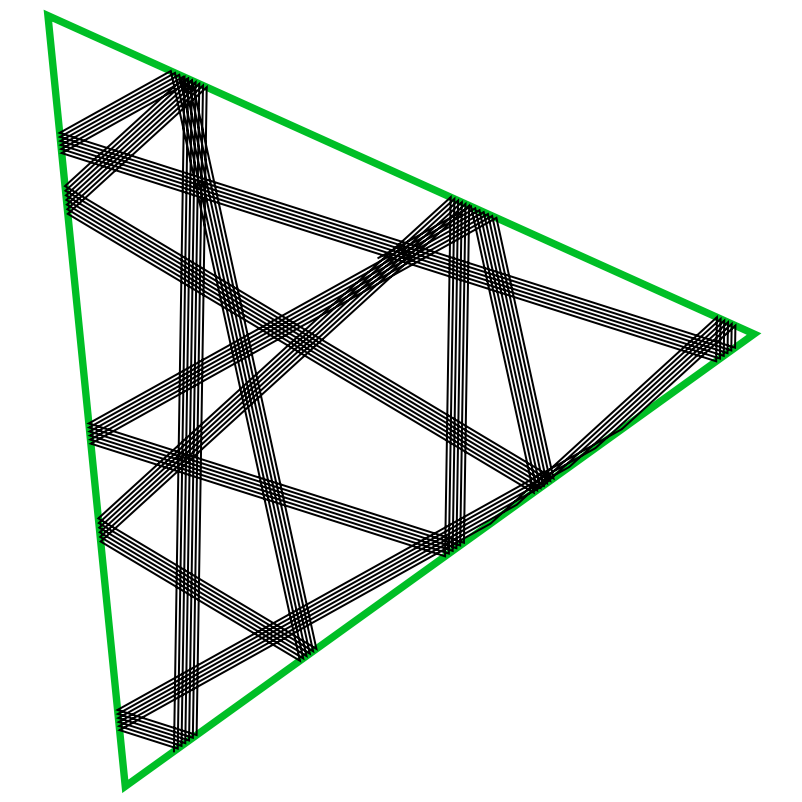15 steps in the evolution for several geometries:

 In:=Out=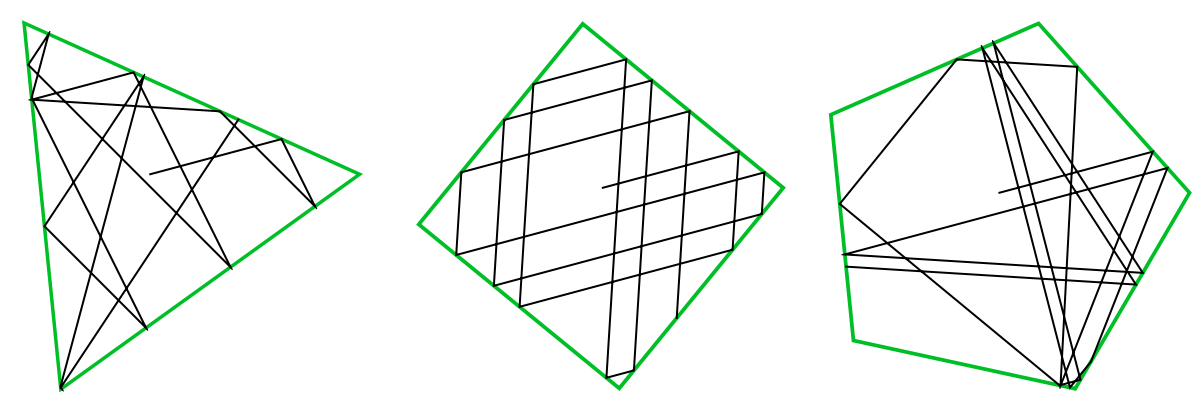Increment gradually the initial position in y-axis:

 In:=Out=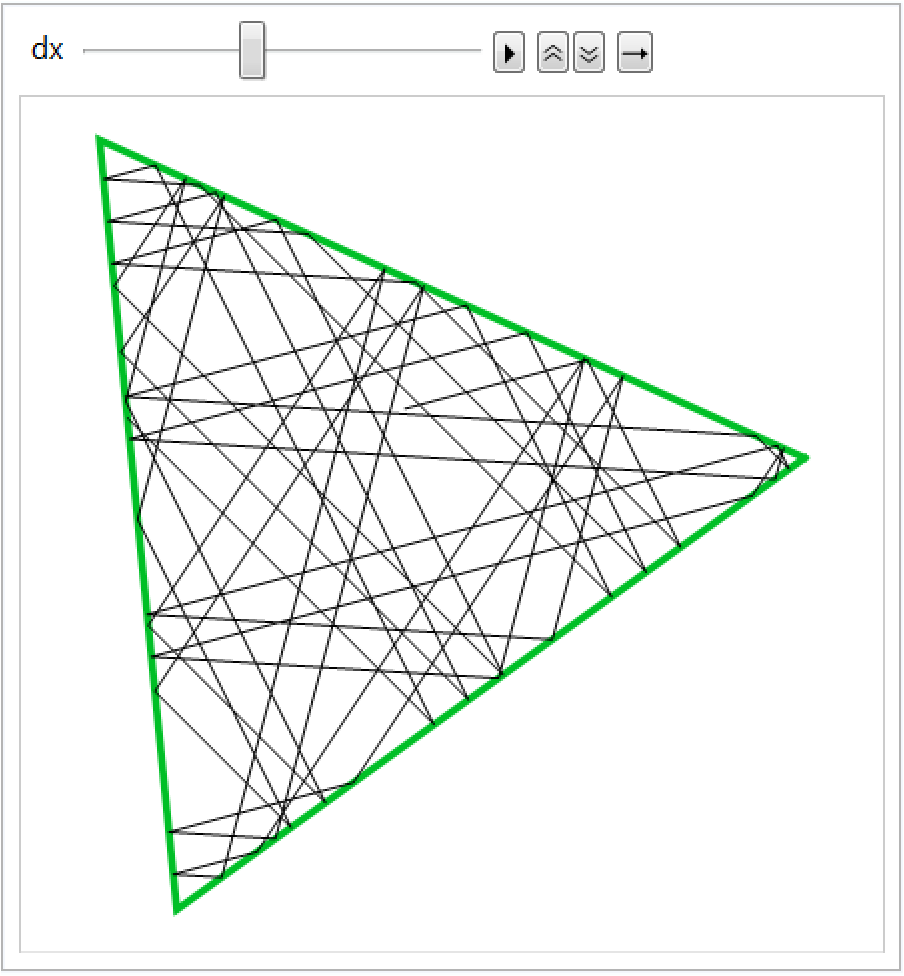A higher number of steps:

 In:=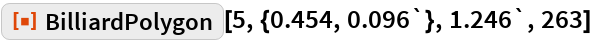Out=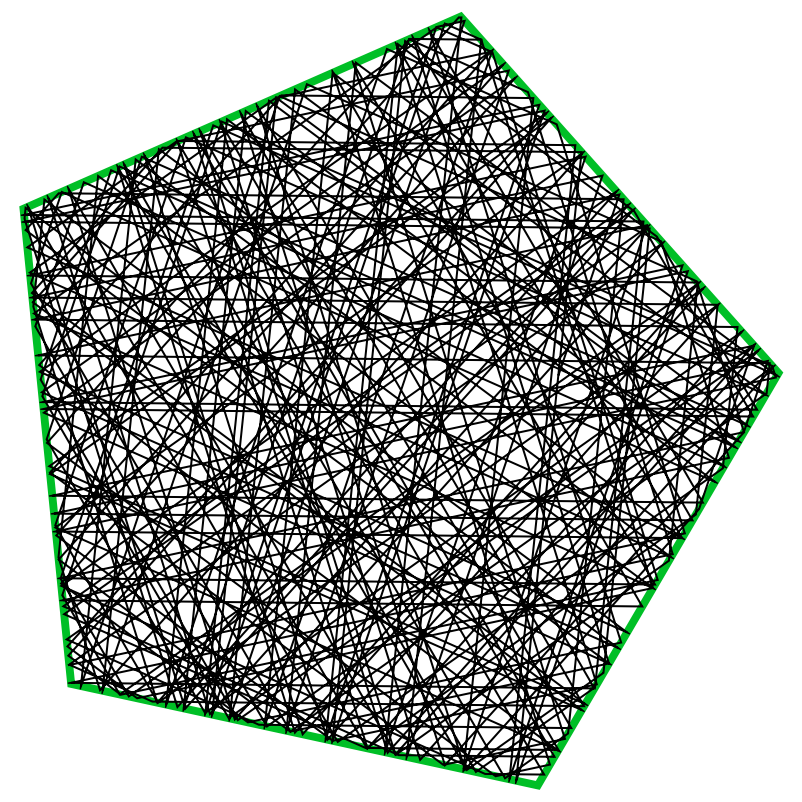## Requirements

Wolfram Language 11.3 (March 2018) or above